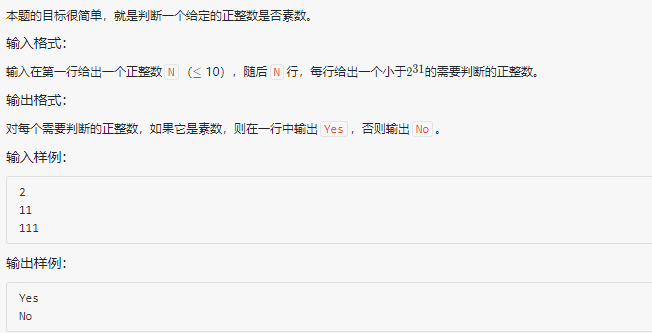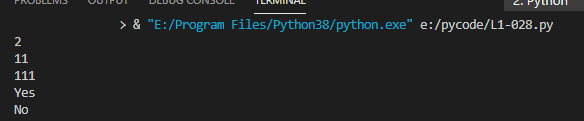• list1 = [] def function(x): if x < 2: #被判读数为1 return False if x==2 or x==3: #被判读数为2或3 return True if x%6 != 1 and x%6 != 5: #被判读数不在6n-1和6n+1数列 retur...list1 = []

def function(x):
if x < 2:       #被判读数为1
return False
if x==2 or x==3:    #被判读数为2或3
return True
if x%6 != 1 and x%6 != 5:   #被判读数不在6n-1和6n+1数列
return False
i = int(5)
while i*i <= x:
if x%i == 0 or x%(i+2) == 0:    #被判读数在6n-1和6n+1数列
return False
else:
i = i+6
return True

n = int(input())
for i in range(n):
list1.append(0)     #空的列表不能直接指定其位置
list1[i] = int(input())
for x in list1:
if function(x) == True:
print("Yes")
elif function(x) == False:
print("No")

运行示例：展开全文算法
• python判断素数程序什么是质数？ (What is a prime number?)A prime number is a natural number that is greater than 1 and cannot be formed by multiplying two smaller natural numbers.质数是大于1的自然数，...
python判断素数程序什么是质数？ (What is a prime number?)A prime number is a natural number that is greater than 1 and cannot be formed by multiplying two smaller natural numbers.质数是大于1的自然数，不能通过将两个较小的自然数相乘而形成。Given a number num, we have to check whether num is a prime number or not.给定数字num ，我们必须检查num是否是质数。Example:例：Input:num = 59Output:59 is a prime numberInput:num = 123Output:123 is not a prime number程序检查Python中的素数 (Program to check prime number in Python)# Python program to check prime number# Function to check prime numberdef isPrime(n):return all([(n % j) for j in range(2, int(n/2)+1)]) and n>1# Main codenum = 59if isPrime(num):print(num, "is a prime number")else:print(num, "is not a prime number")num = 7if isPrime(num):print(num, "is a prime number")else:print(num, "is not a prime number")num = 123if isPrime(num):print(num, "is a prime number")else:print(num, "is not a prime number")Output输出量59 is a prime number7 is a prime number123 is not a prime number翻译自: https://www.includehelp.com/python/check-prime-number.aspxpython判断素数程序
展开全文• 判断一个给定的正整数是否素数输入格式:输入在第一行给出一个正整数n(≤ 10)，随后n行，每行给出一个小于.../usr/bin/python# -*- coding: utf-8 -*-#定义一个判断单个素数的方法def sus(n):v = trueif n == 1:v...
判断一个给定的正整数是否素数输入格式:输入在第一行给出一个正整数n(≤ 10)，随后n行，每行给出一个小于1000000 的需要判断的正整数输出格式:对每个需要判断的正整数，如果它是素数，则在一行中输出yes，否则输出no代码如下：#!/usr/bin/python# -*- coding: utf-8 -*-#定义一个判断单个素数的方法def sus(n):v = trueif n == 1:v = falsefor i in range(2,n):if n%i == 0:v = falsebreakif v:return "yes"else:return "no"n = int(input())l = list()for i in range(0,n):s = int(input())l.append(s)for i in range(0,len(l)):print(sus(l[i]))这个程序不难，知道素数怎么求就行。对于类似于这种的判断一个数是什么数，需要处理很多的。都可以定义一个方法。1、定义一个方法求素数(什么数都可以)2、然后循环判断，输出结果。题外话：很多情况下都可以先定义一个新列表，然后append添加数据。最后在打印。目前我是爱上了这种方法。转自：https://www.cnblogs.com/Renqy/p/12736261.html如您对本文有疑问或者有任何想说的，请点击进行留言回复，万千网友为您解惑！
展开全文• 一个大于1的自然数，除了1和它本身外，不能...首先我们来第一个传统的判断思路：def handlerNum(num):# 质数大于 1if num > 1:# 查看是否有其他因子for i in range(2, num//2+1):if (num % i) == 0:print(num,"不...
一个大于1的自然数，除了1和它本身外，不能被其他自然数(质数)整除(2, 3, 5, 7等)，换句话说就是该数除了1和它本身以外不再有其他的因数。首先我们来第一个传统的判断思路：def handlerNum(num):# 质数大于 1if num > 1:# 查看是否有其他因子for i in range(2, num//2+1):if (num % i) == 0:print(num,"不是质数")breakelse:print(num, "是质数")# 如果输入的数字小于或等于 1，不是质数else:print(num, "不是质数")if __name__ == '__main__':# 用户输入一个数字num = int(input("请输入一个数字: "))# 调用函数处理方法handlerNum(num)其实上面循环中的else和if并不是成对的，而是和for并排的，当然for和else搭配出现并不少见，慢慢地会有所体会的，这段代码的含义是，当for里面的条件都不满足时，就会执行else里面的代码。以上就是我们按照传统的思路来解题，其实还有一种更快，更简单的方法解题，那就是利用真或假来判断。#处理函数def IsPrime(num):#根据质数的定义，其必须大于0if num == 1:return False#循环需要判断的次数for i in range(2, num // 2 + 1):#如果该数有其他的因子返回False，即不是质数if num % i == 0:return Falsereturn Trueif __name__ == '__main__':#输入一个数字num = eval(input("请输入一个数，判断是否为质数："))#调用方法(如果是质数返回True，否则返回False)，打印结果print(IsPrime(num))这两种方法大体都差不多，只不过这给我们以后解决问题提供了一种新的思路。以上这篇Python 判断是否为质数或素数的实例就是小编分享给大家的全部内容了，希望能给大家一个参考，也希望大家多多支持我们。本文标题: Python 判断是否为质数或素数的实例本文地址: http://www.cppcns.com/jiaoben/python/209357.html
展开全文• 质数(Prime number)，又称素数，指在大于1的自然数中，除了1和该数自身外，无法被其他自然数整除的数(也可定义为只有1与该数本身两个因数的数)。那么想计算出一个随机数是不是质数用Python应该怎么写呢？首先第一句...
• 来看这一种判断素数（质数）的函数：form math import sartdef is_prime(n):if n==1:return Falsefor i in range(2, int(sqrt(n) + 1)):if n % i == 0:return Falsereturn True看起来，这是一种比较优秀的方法了，...
• 使用Lucas-Lehmer primality test来检查什么素数产生梅森素数(Mp)，这样就避免了检查梅森数本身的素性，因为它们非常大，而且只有素数指数产生Mp，通过这个测试，你只需要p是素数并通过这个测试，那么你就可以把你的...
• 今天咱们继续来学一个python小例子，其实在上一篇的ghpython中有学过，就是判断一个自然数是否是素数，如果是输出为True，如果不是，输出为否。这么简单的一个问题，今天为啥又要单独拿出来讨论呢，因为对于之前的...
• 本文实例讲述了python实现求最大公约数及判断素数的方法。分享给大家供大家参考。具体实现方法如下： #!/usr/bin/env pythondef showMaxFactor(num):count = num / 2while count > 1:if num % count == 0:print '...
• 如果一个数字能表示成 p^q，且p是一个素数，q为大于1的正整数，则此数字就是超级素数幂。param number: 测试该数字是否是超级素数幂return: 如果不是就返回 False，如果是就返回 p 和 q 值例如，输入125，返回(5，3)...
• 来看这一种判断素数（质数）的函数：form math import sartdef is_prime(n):if n==1:return Falsefor i in range(2, int(sqrt(n) + 1)):if n % i == 0:return Falsereturn True看起来，这是一种比较优秀的方法了，...
• 质数（Prime number），又称素数，指在大于1的自然数中，除了1和该数自身外，无法被其他自然数整除的数（也可定义为只有1与该数本身两个因数的数）。那么想计算出一个随机数是不是质数用Python应该怎么写呢？首先第...
• 1 #运用python的数学函数23 importmath45 defisPrime(n):6 if n
• python判断素数程序 什么是质数？ (What is a prime number?) A prime number is a natural number that is greater than 1 and cannot be formed by multiplying two smaller natural numbers. 质数是大于1的...算法 tensorflow 运维
• 素数的定义：一个数如果只能被1和自身整除，则该数为素数。如果用编程的思维思考，则是说，如果一个数被比它小的数（1除外）整除后余数为0，则说明这个数可以被其他数整除，则该数为合数，否则该数为质数。Python...
• 在本篇文章里小编给大家整理了关于python判断素数的方法和代码，需要的朋友们可以学习下。
• 今天咱们继续来看老潘微博里的一个python小案例。案例讨论的是可逆素数，所谓可逆素数，就是说一个数本身是素数，而将其各位数字逆序反过来所构成的数字仍然是素数。利用int和str的相互转换，可以快速实现将int型...
• 判断n＋1个数最小值m=int(input())i=0n=int(input())while ij: m=jprint(m)＃求完全立方数立方根Number = int(input(“请输入一个完全立方数”))i = 0while i < = Number:if i*i*i == Number: print(“立方根.....
• 第一种方法利用这个素数的定义，我们可以得出第一种判断素数的方法：int isPrime1(int n){int i = 0;//2是素数if(n <= 3)return n > 1;//当n不能被除了1和n自身整除的数外的数是素数for(i = 2; i < n; i ++...
• 判断素数的两种方法 def prime(n): for i in range(2,n): if n%i ==0: return False else: return True from math import sqrt,pow def prime(num): """检测num是不是质数""" ...
• python判断素数程序This program will check whether a given number is Prime or Not, in this program we will divide the number from 2 to square root of that number, if the number is divided by any number...java 编程语言
• 【本文结构】题目信息：来源、地址、序号、描述题目答案：简要分析，...来源、地址、序号、描述【题目信息】来源：菜鸟教程地址：https://dwz.cn/Ol2QUMGD序号：12描述：判断101-200之间有多少个素数，并输出所有素...
• 先来看下什么是质数：质数(Prime number)，又称素数，指在大于1的自然数中，除了1和该数自身外，无法被其他自然数整除的数(也可定义为只有1与该数本身两个因数的数)。简单来说就是，只能除以1和自身的数(需要大于1)...
• 给定一个大于2的正整数n，打印出小于n（不包括n且n不大于100）的所有素数。 要求将符合条件的输出填入一个列表中，打印的结果为该列表。 （素数：只能被1和它本身整除的...#判断素数函数 def num(a): for i in rang......

# 判断素数pythonpython 订阅